| Technical Writer: Vedant Mane & Thilakraj Devadiga | Technical Review: ABCOM Team | Level: Intermediate | Banner Image Source : Internet |

# Motivation:

In today’s world, we see a lot of AI&ML research applied to healthcare and Medical sciences. ML and AI can help diseased persons and medical practitioners in various ways. The most popular usage of machine learning is in automating pharmaceutical billing, clinical verdict support, and the improvement of clinical care guidelines. There are many types of research and examples of ML applied in healthcare. In radiology, deep learning has helped in classifying complex patterns observed in CT, MRT, PET scans. Such ML models almost match the skills of an experienced radiologist. Google developed an AI model with 89% accuracy at par with the radiologists to detect breast cancer. As we are talking about breast cancer, it is the second foremost cause of death in females worldwide. If a doctor diagnosed a person with a tumor, the first step the doctor will take is to check whether the Tumor is malignant (cancerous) or benign (non-cancerous) to decide on his treatment plan.
In this tutorial, I will show you several EDA (Exploratory Data Analysis) techniques to explore the data collected for diagnosis of breast cancer.

# Application

We will use the dataset provided in UCI machine learning repository for demonstrating EDA. The dataset includes features computed from a digitized picture of a fine needle aspirate of a breast mass. These features describe the characteristics of the tumor cell nuclei present in the digitized image. Our goal is to diagnose if a data point shows a malignant or benign tumor. The dataset contains a huge number of features. You will learn the feature selection strategy to minimize this set. For exploring and feature selection, you will use several visualization techniques such as box plot, violin plot, correlation matrix, and Swarm Plot.

# Project:

Create a new Colab project and rename it to Breast-cancer Diagnosis.

Import the following packages:

``````import numpy as np
import pandas as pd
import seaborn as sns
import matplotlib.pyplot as plt
import time
import warnings
from sklearn.model_selection import train_test_split
from sklearn.ensemble import RandomForestClassifier
from sklearn.metrics import confusion_matrix,classification_report
warnings.filterwarnings("ignore")
``````

We will be using the `RandomForestClassifier` from sklearn to classify the data into two categories - malignant or benign.

We have uploaded the dataset on our GitHub for your quick access in the Colab project. Load the data into your project using the following command:

``````data = pd.read_csv("https://raw.githubusercontent.com/abcom-mltutorials/breast-cancer-data/main/data.csv" , index_col = 0)
``````

Examine few records of the loaded data:

``````data.head()
``````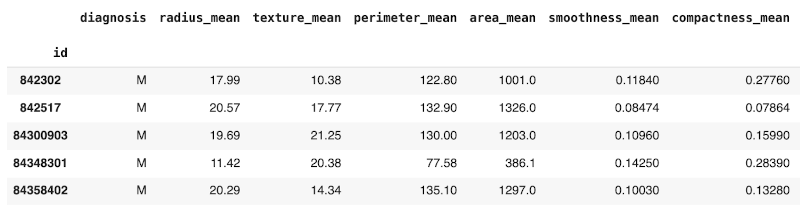Check the dataset dimensions - there are 569 data points and 32 features, a very large number to explore. For a complete description of the features, we refer you to the original source of the dataset. Training the model for so many features would be expensive in terms of time and resources. We need to identify the features which have more prominence in cancer detection.

First, we will separate out the target variable from the dataset.

``````y = data.diagnosis
drop_cols = ["Unnamed: 32", "diagnosis"]
x = data.drop(drop_cols, axis = 1)
``````

We now start exploring the features dataset.

## Exploratory Data Analysis

For analysis, we will use several visualization procedures. We will review the plots to understand the patterns, point anomalies and testing/validating our assumptions. We begin by checking if the dataset is balanced.

### Balanced/Unbalanced Sets

We check if there is a balance between the malignant and benign data points. To do this, we plot the target count for each using the following code:

``````ax = sns.countplot(y, label = "Count")
``````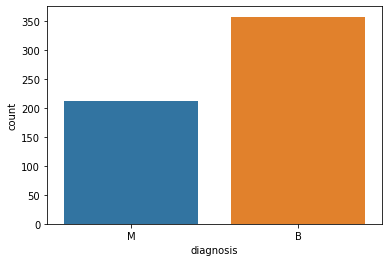You may also print the counts using following code:

``````B, M = y.value_counts()
print("Number of Benign Tumor: {}\nNumber of Malignant Tumors: {}".format(B,M))
``````

This is the output:

``````Number of Benign Tumors : 357
Number of Malignant Tumors :  212
``````

We conclude that the dataset is well balanced.

## Feature Value Statistics

We now generate some statistics on each column value to understand its distribution. Calling `describe` method provides you with the full statistics like max, min, standard deviation, mean/average and percentile.

``````x.describe()
``````

Output: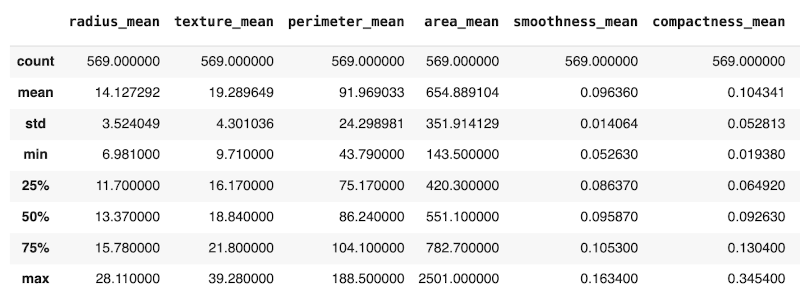## Visualizations

The best way to understand the data better is to plot it. We use the Seaborn visualization library for this purpose. Written on top of Matplotlib, it provides a high-level interface for drawing attractive and informative statistical graphics.

First, we will do violin plots.

### Violin Plots

A Violin Plot shows the distribution of the data and its probability density. This chart is a combination of a Box Plot and a Density Plot that is rotated and placed on each side, to show the distribution shape of the data. The plot for all the features in our dataset is generated using the following code fragment.

``````data = x
data_std = (data - data.mean()) / data.std()
data = pd.concat([y, data_std.iloc[:, 0 : 30]], axis = 1)
data = pd.melt(data, id_vars="diagnosis",
var_name = "features",
value_name = "value")
plt.figure(figsize = (20,10))
sns.violinplot(x="features", y = "value", hue = "diagnosis",
data = data, split = True, inner = "quart")
plt.xticks(rotation = 45)
``````

This is the output: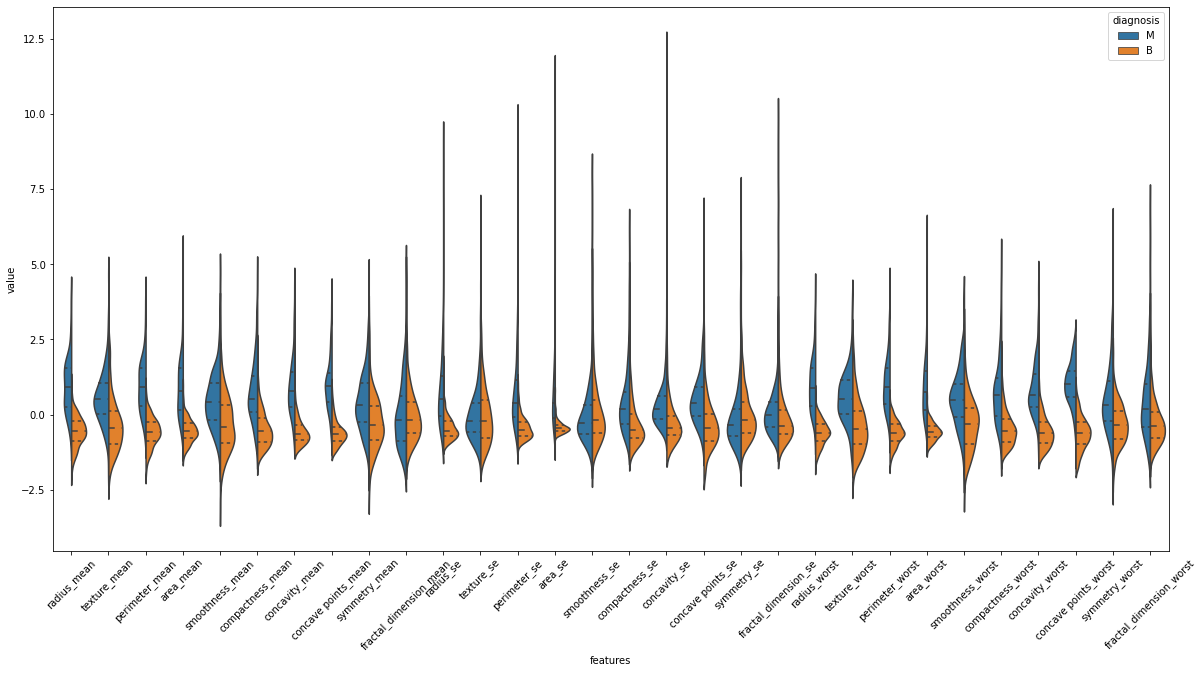The plot appears to be cluttered and thus is hard to interpret. To make it easier to understand, we will plot three independent violin plots with ten features each.

Let's first understand what this figure means and how we can interpret it.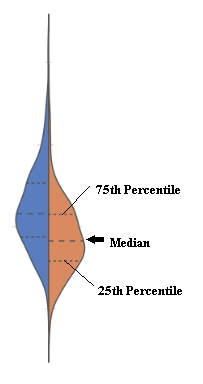In this figure, each side of the vertical line shows data distribution for both the target classes B (orange) and M (blue) for a particular feature. The horizontal lines represent different quartiles like in a box plot. The advantage of a violin plot over a box plot is that we can directly compare both classes instead of creating multiple box plots for each target label.

We will now generate a plot for the first ten features.

### Plotting First Ten Features

To plot only the first ten features, you pick first data points using indexing. The rest of the plotting code remains the same.

``````data = pd.concat([y, data_std.iloc[:, 0 : 10]], axis = 1)
data = pd.melt(data, id_vars="diagnosis",
var_name = "features",
value_name = "value")
plt.figure(figsize = (20,10))
sns.violinplot(x="features", y = "value", hue = "diagnosis",
data = data, split = True, inner = "quart")
plt.xticks(rotation = 45)
``````

Output: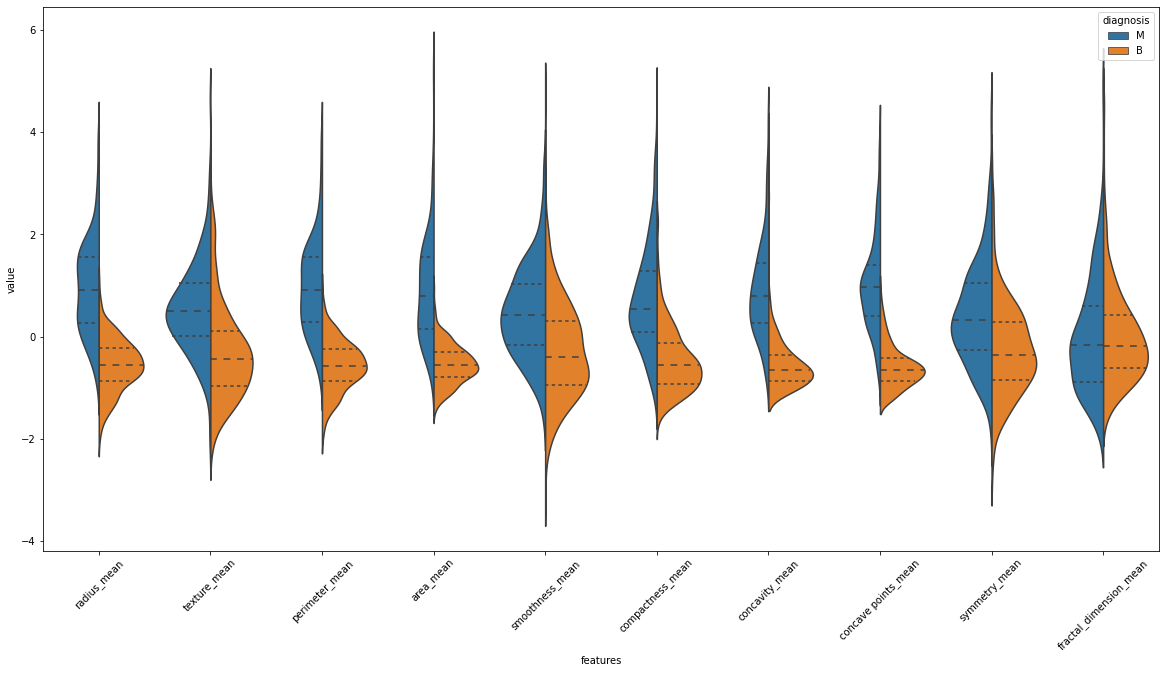You can now interpret these results better. For example, we see that the median of texture_mean column looks separated, which means it might be an excellent feature. While in the fractal_dimension_mean column, the medians are very close to each other, so data is similar for the Malignant and Benign.

Next, examine features 11 through 20.

### Plotting Next 10 Features

Just change the index in the dataframe.

``````data = pd.concat([y, data_std.iloc[:, 10 : 20]], axis = 1)
``````

This is the output: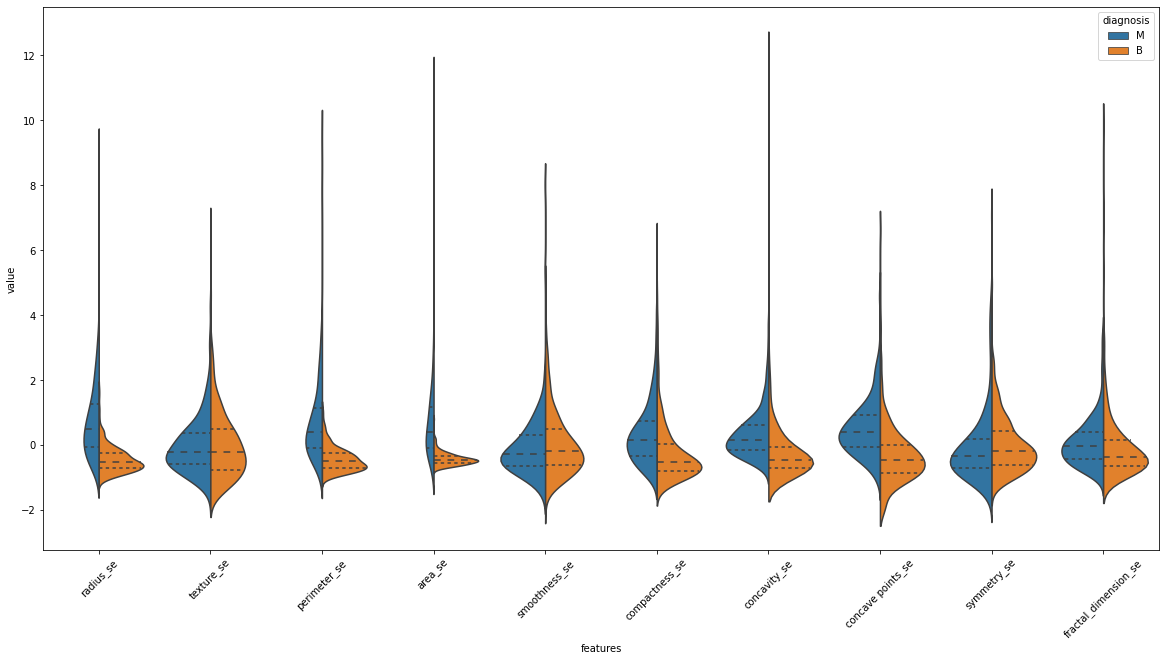The medians for classes vary little for the standard error features above, except for the concave points_se and concavity_se feature. The smoothness_se or symmetry_se have a very similar distribution which could make classification using this feature difficult.

## Plotting Last 10 Features

Modify the index entry as follows:

``````data = pd.concat([y, data_std.iloc[:, 20 : 30]], axis = 1)
``````

This is the output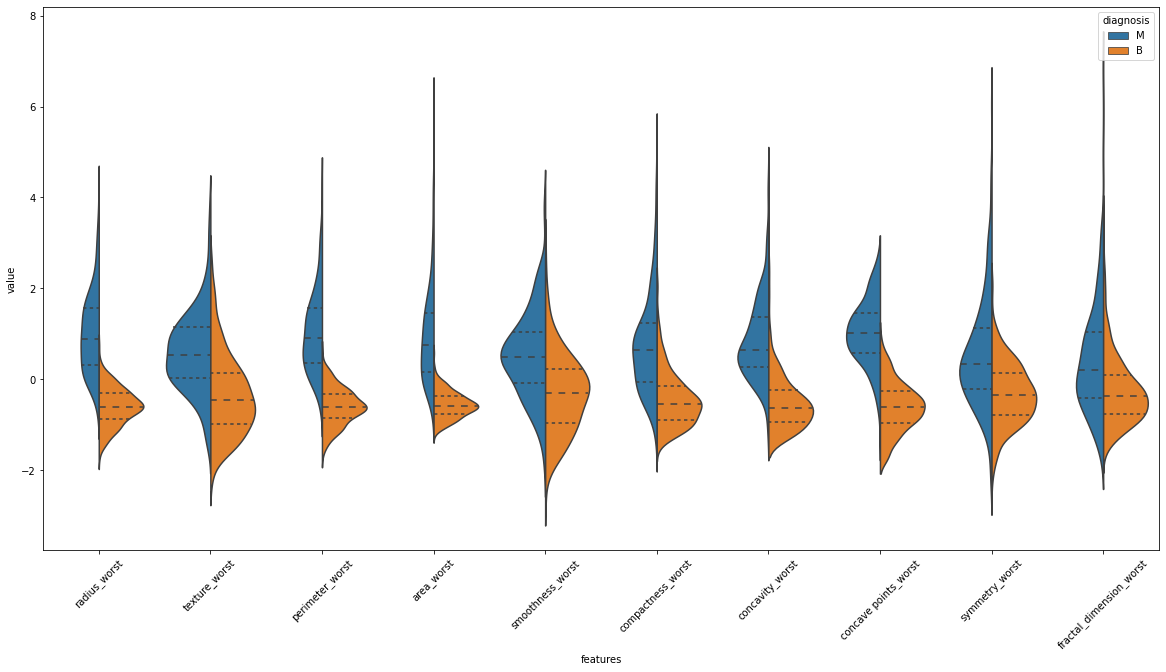The area_worst looks well separated, so it might be easier to use this feature. The concavity_worst and concave_points_worst seem to have a comparable data distribution.

After examining all thirty features for the distribution, we will show you how to generate a comparative box plot.

# Comparative Box Plot

We generate a box plot by calling the `boxplot` method of the sns library. We will generate the plots for both values of the target - malignant and benign.

``````plt.figure(figsize = (15,10))
sns.boxplot(x = "features", y = "value", hue = "diagnosis", data = data)
plt.xticks(rotation = 45)
``````

This is the output: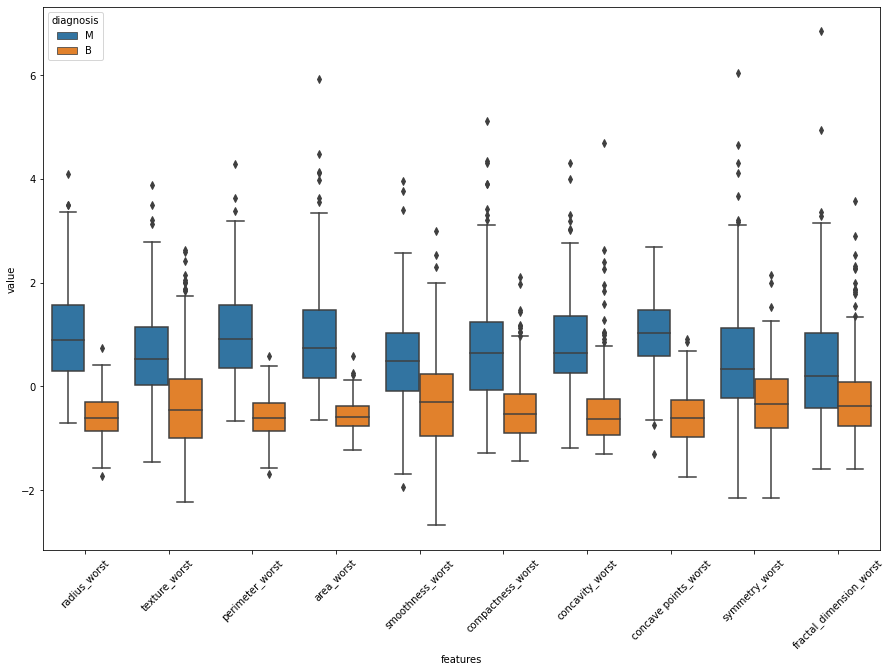You can easily see that it is a lot more helpful to use violin plots as compared to box plots for exploring data. Now, we will show you another kind of plot for data analysis.

## Swarm Plot

We can study a swarm plot on its own. It is an excellent complement to a box or violin plot when you want to show all observations along with some representation of the underlying distribution. The swarm plot is like a strip plot. The only difference is that we adjust the points so that they do not overlap. This gives us a better representation of the distribution of values. Similar to violin plot, we will create this plot in batches to avoid the cluttering of features.

### Plot for First 10 Features

Here is the code for swarm plot:

``````sns.set(style="whitegrid", palette="muted")
data = x
data_std = (data - data.mean()) / data.std()
data = pd.concat([y, data_std.iloc[:, 0 : 10]], axis = 1)
data = pd.melt(data, id_vars="diagnosis",
var_name = "features",
value_name = "value")
plt.figure(figsize = (15,10))
sns.swarmplot(x="features", y = "value", hue = "diagnosis", data = data)
plt.xticks(rotation = 45)
``````

This is the output: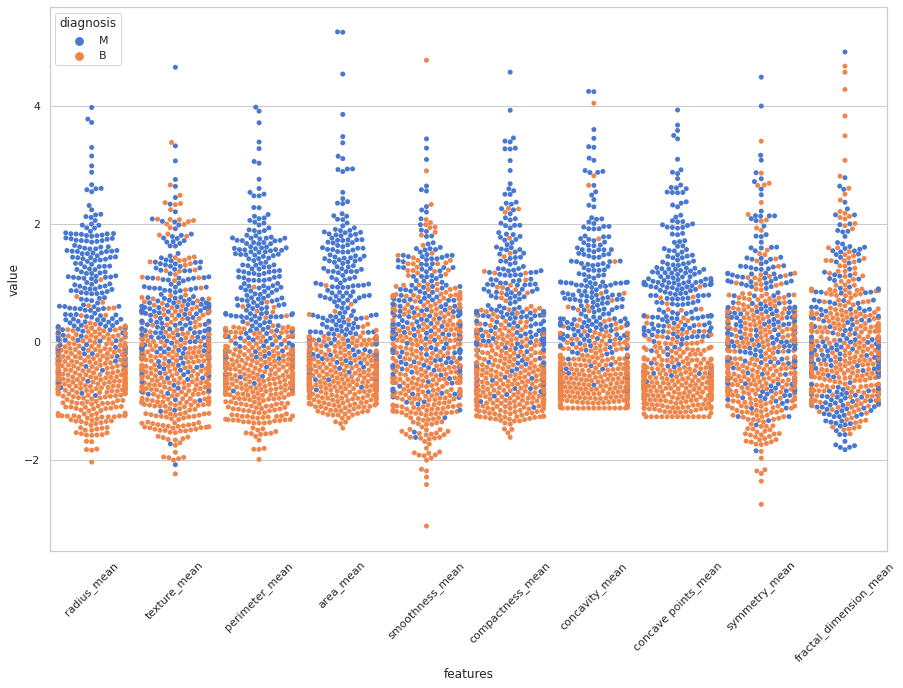From this plot, we can understand how the underlying data gets distributed for certain features regarding the target label. For example, look at the radius_mean and fractal_dimension_mean features for both target labels, Benign (orange) and Malignant (blue). In radius_mean for both the classes, the distribution is in a different range except for a few data points. While in fractal_dimension_mean, many values are in a similar distribution. Same is the case for symmetry mean and smoothness_mean features.

Likewise, generate swam plots for the remaining two ranges and study the data distribution across two classes for each of the features.

I will now show you how to use a correlation plot for observing correlation between different features.

## Pairwise Correlations

To observe the correlation between different features, you use a correlation plot.

### Correlation Matrix

A correlation matrix is a table showing correlation coefficients between variables. Each cell in the table shows the correlation between two variables. A correlation matrix is used to summarize data, as an input or diagnostics into an advanced analysis.You create the matrix plot by calling the heatmap method

``````f, ax = plt.subplots(figsize = (18,18))
sns.heatmap(x.corr(), annot = True, linewidth = .5, fmt = ".1f", ax = ax)
``````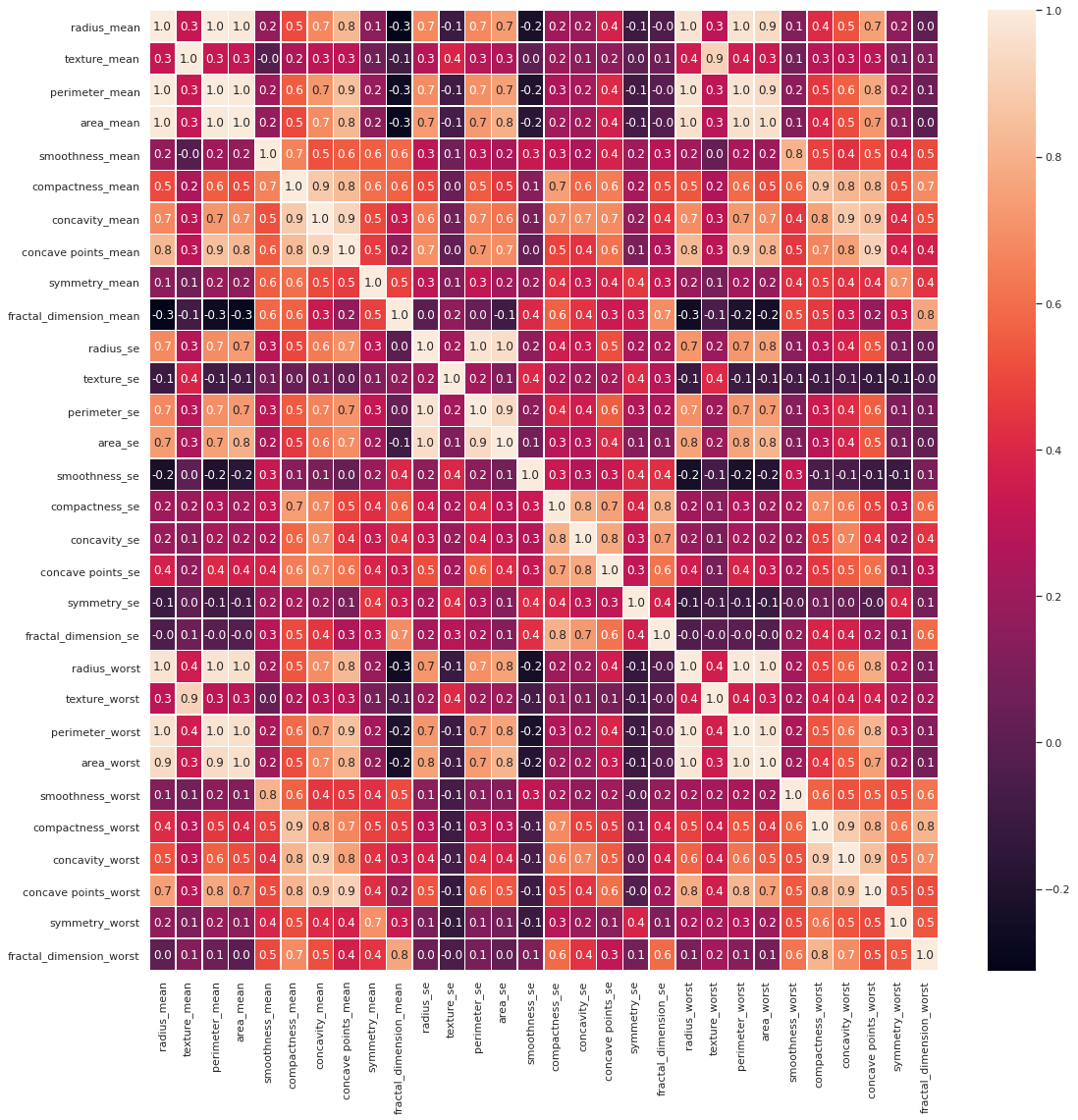All boxes have a certain figure embedded in it. This figure defines the correlation between the two columns (features) shown by the row and column names. A large value for this figure shows that the two features have a very strong correlation with each other. Let us now examine some of these correlations to explore our dataset.

In our plot, we can observe that the means, standard errors, and worst dimension lengths of compactness, concavity, and concave points of tumors are highly correlated amongst each other (correlation > 0.8). Then the mean, standard errors, and worst dimensions of radius, perimeter, and area of tumors have a correlation of 1. The texture_mean and texture_worst have a correlation of 0.9, area_worst and area_mean have a correlation of 1.

Thus, in this matrix, we observe that there are a good number of features, which are highly correlated. As the correlated features do not add any value to machine learning, we will drop one from each pair. For dropping, we will use the criterion of having the correlation index value greater than 0.9.

``````drop_cols = ["perimeter_mean", "radius_mean", "compactness_mean",
"concave points_worst", "compactness_se", "concave points_se",
"texture_worst", "area_worst"]
df = x.drop(drop_cols, axis = 1)
``````

This is the output: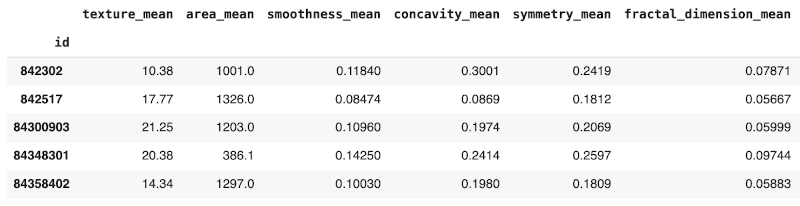We plot the correlation matrix for our new set of features.

``````f, ax = plt.subplots(figsize = (16,16))
sns.heatmap(df.corr(), annot = True, linewidth = 0.5, fmt = ".1f", ax = ax)
``````

This is the output: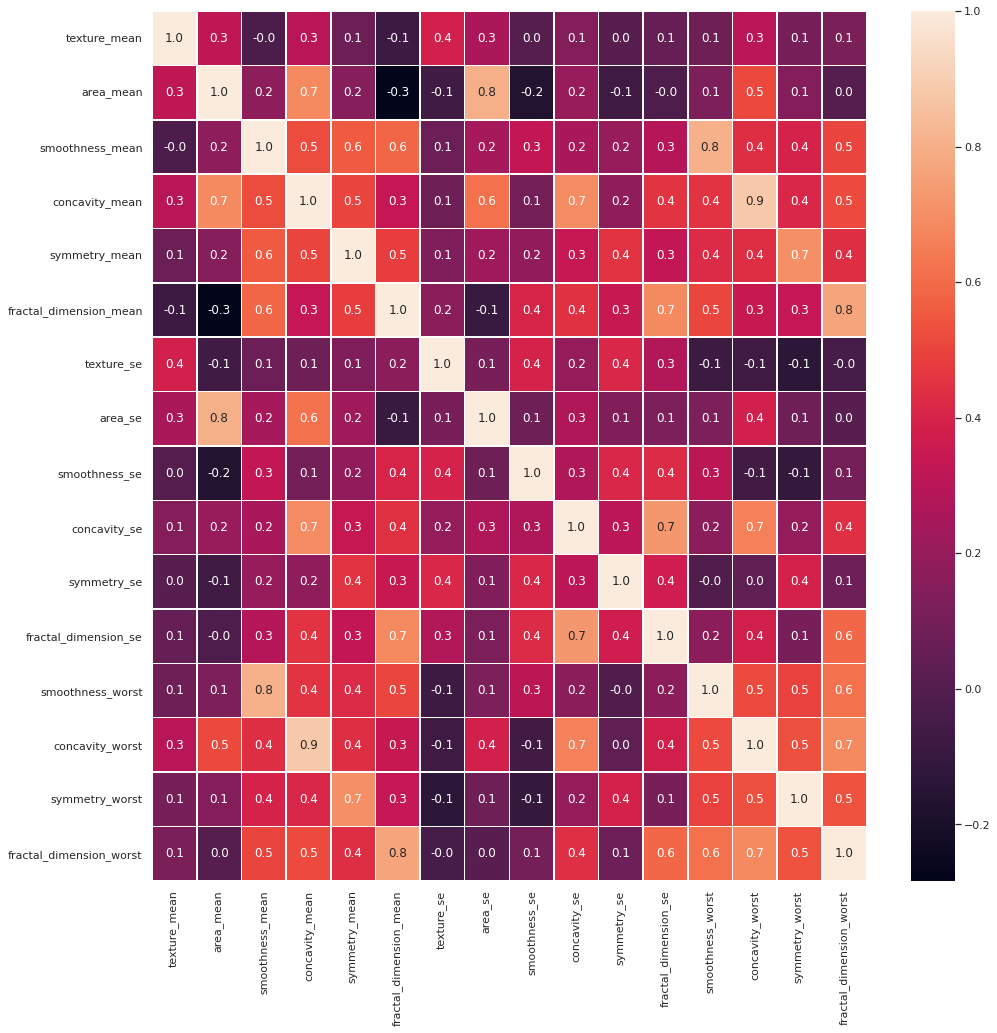Now there are no pairs with a correlation of 0.9 in the features set.

We now prepare our dataset for training and testing.

# Training/Testing Datasets

We split the dataset into training and testing using the following statement:

``````X_train, X_test, y_train, y_test = train_test_split(df, y, test_size = 0.2, random_state = 42)
``````

We reserved 20% of data for testing.

At this point, we are done with data preparation, so let us proceed to model building.

# Model Building

For the classification task, we will use one of the popular algorithms, Random Forest. We will initialize the model with some minimal model parameters.

``````model = RandomForestClassifier(n_estimators = 10, n_jobs = -1, criterion = "entropy", max_features = "auto", random_state = 1234)
model.fit(X_train, y_train)
``````

After we initialize the model, we are ready to perform the actual training operation on the model by calling the fit function along with the training sets as its arguments.

``````model.score(X_test, y_test)
``````

After the model is trained, we evaluate its performance.

# Model Evaluation

We estimate the generalization accuracy of a model on unseen data by checking the model’s accuracy score.

``````0.9824561403508771
``````

We can see that the model has given a 98% accuracy, which is impressive. We will now do some analysis of the results.

## Result Analysis

We will first plot the confusion matrix. We write a function for plotting the confusion matrix as follows:

``````# function to visualize confusion matrix
def conf_matrix(cm):
plt.clf()
plt.imshow(cm, interpolation='nearest', cmap=plt.cm.Wistia)
classNames = ['B','M']
plt.title('Harmerful or Not')
plt.ylabel('True label')
plt.xlabel('Predicted label')
tick_marks = np.arange(len(classNames))
plt.xticks(tick_marks, classNames)
plt.yticks(tick_marks, classNames)
for i in range(2):
for j in range(2):
plt.text(j,i,str(cm[i][j]))
plt.show()
``````

We will ask our trained model to predict the test dataset and then compare its results with the actual values using a confusion matrix.

``````y_pred=model.predict(X_test)
cm=confusion_matrix(y_test,y_pred)
conf_matrix(cm)
``````

This is the output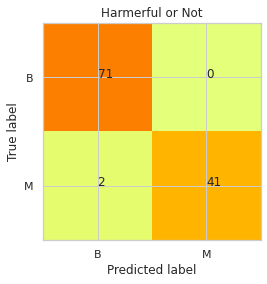We can observe from the plot that only two values were wrongly predicted from the test dataset.

Next, we will create a classification report.

## Classification Report

We create a classification report by calling an in-built function.

``````print(classification_report(y_test,y_pred))
``````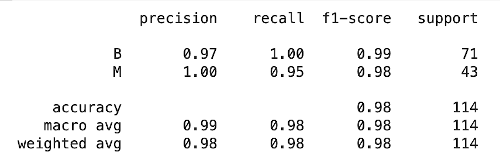The classification report displays the precision, recall, F1, and support scores for the model. As you know, the precision which is a ratio of true positives to the sum of true and false positives, gives us a measure of classification accuracy. The Recall, which is the ratio of true positives to the sum of true positives and false negatives, shows us the classifier’s ability to correctly identify all positives in the data. F1 score is a weighted harmonic average of precision and recalls. The most favorable score is 1.0, and the worst is 0.0. The weighted average score analyzes/compares various classifier models. The Support is the number of occurrences of the label in the specified dataset.

Now, we will show you how to determine the features which have played a prominent role in the model training. You may then improve your model further by taking a revised version of the dataset.

Finally, I will show you how to evaluate the importance of the various features in model’s predictions.

# Feature Importance

Tree-based Algorithms do calculate feature importance while training a model. By using the `feature_importances_` attribute of `RandomForestClassifier`, we can plot the feature importance on our data.

``````plt.figure(figsize=(8,6))
n_features = len(X_train.columns)
plt.barh(range(n_features),model.feature_importances_, align='center')
plt.yticks(np.arange(n_features), X_train.columns)
plt.xlabel("Feature importance")
plt.ylabel("Feature")
plt.ylim(-1, n_features)
``````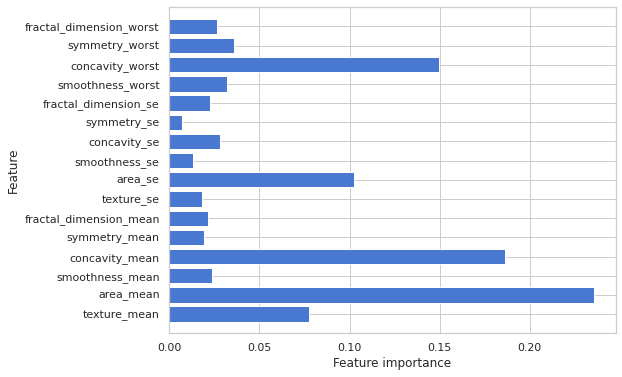As you can see, the model gave the highest importance to the area_mean feature, followed by concavity_mean and then concavity_worst.

# Conclusion

In this project, you learned how EDA helps in feature selection for any machine learning task.This selection helps in shrinking your features collection to enable faster and more accurate training. You applied these techniques to a critical Medical Sciences field, where the stakes are high and mistakes are not mostly accepted. Good luck!